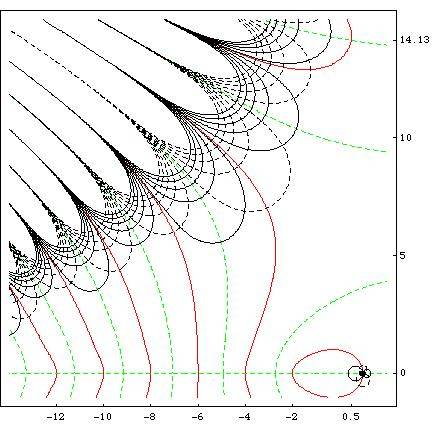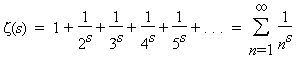# The Most Beautiful Equation?

Lets elect World's most beautiful equation!

Two categories:

1.Mathematics
2.Physics

My personal favourites would be:

1. e$$^{i\pi}$$+1=0 (Do i need to give an argument?)

2. E=mc$$^{2}$$ (I know its mainstream.. but i doesent get much more simple and general than this!)

Last edited:

$$i\hbar\frac{\partial\Psi}{\partial t} = \frac{\hbar^2}{2m}\frac{\partial^2\Psi}{\partial x^2} + V\Psi$$

vanesch
Staff Emeritus
Gold Member
I'd say: 1 = 0. From this one, you can derive everythingMaxwell's equations of EM?

$$\nabla \cdot D= \rho$$
$$\nabla \cdot B=0$$
$$\nabla \times E=- \partial B/ \partial t$$
$$\nabla \times H=J+ \partial D/ \partial t$$

$$p = \frac{h}{\lambda}$$

robphy
Homework Helper
Gold Member
$$i\hbar\frac{\partial\Psi}{\partial t} = \frac{\hbar^2}{2m}\frac{\partial^2\Psi}{\partial x^2} + V\Psi$$

I think you are missing a minus sign.

1/. cos²(x) + sin²(x) = 1
2/. ω² = k/m

Borek
Mentor
2. E=mc$$^{2}$$ (I know its mainstream.. but i doesent get much more simple and general than this!)

Isn't it only an approximation?FD=½ρv2ACD

Some engineering fudgeamatics

Last edited:
Binhjuventus™
For me, it's:
i2=-1

Isn't it only an approximation?It's true http://nz.news.yahoo.com/a/-/world/5160859/einsteins-emc2-proven-103-years/ [Broken]

Last edited by a moderator:
Borek
Mentor
As far as I remember mc2 is only a first term of the power series. Next terms are smaller by at least c2 factor (or even c4, my memory fails me here), so they can be safely ignored, but E=mc2 is still only an approximation.

As far as I remember mc2 is only a first term of the power series. Next terms are smaller by at least c2 factor (or even c4, my memory fails me here), so they can be safely ignored, but E=mc2 is still only an approximation.

I think you refer this equation:

E2=m2c4+p2c2

robphy
Homework Helper
Gold Member
As far as I remember mc2 is only a first term of the power series. Next terms are smaller by at least c2 factor (or even c4, my memory fails me here), so they can be safely ignored, but E=mc2 is still only an approximation.

You may referring to the Taylor expansion (with respect to the velocity) for the relativistic energy:
http://en.wikipedia.org/wiki/Kinetic_energy
expressed as
$$E_{rel}=m_{rel}c^2=m_0c^2 \frac{1}{\sqrt{1-(v/c)^2}}=m_0c^2\bigg(1+\frac{1}{2}(v/c)^2+\frac{3}{8}(v/c)^4+\ldots\bigg) \approx m_0c^2 \bigg( 1+\frac{1}{2}(v/c)^2 \bigg)\mbox{[for small (v/c)]}$$

The rest energy $$E_0=m_0c^2$$ is a Lorentz invariant, and $$E_{rel}$$ and $$m_{rel}$$ are observer-dependent quantities.

From a special-relativistic viewpoint, these are exact relations.

From a Newtonian-physics viewpoint, one often refers to some of these terms as "relativistic corrections".

E= ir !

$$i\hbar\frac{\partial\Psi}{\partial t} = - \frac{\hbar^2}{2m}\frac{\partial^2\Psi}{\partial x^2} + V\Psi$$

Well, it may be hard to say. I am a junior in high school, so my physics knowledge is limited, however, I am fond of

i^2 = -1

Imaginary x Imaginary = Real. Seems silly but awesome.

Hayley

Maxwell`s velocity distribution formula

f(v) = 4 $$\pi$$ [ m / 2 $$\pi$$ k T ]^(3/2) v^2 e^(-m v^2 / 2 k T )

The elegance because he derived this formula just by logical reasoning
almost without calculus or any kind of information. Intellectual wizardy.

Last edited:
zeta(s)=1+(2^-s)+(3^-s)+(4^-s)+...

- the zeta function from which the Riemann hypothesis derives.

$$E=mc^2$$.#### Attachments

0! = 1

I don't know if it is beautiful; but it is pretty weird.

The equation relating primes to zeta zeros:

$$\psi(x)=-\frac{1}{2\pi i}\mathop\int\limits_{c-i\infty}^{c+i\infty} \frac{\zeta'(s)}{\zeta(s)}\frac{x^s}{x}ds$$

The equation relating primes to zeta zeros:

$$\psi(x)=-\frac{1}{2\pi i}\mathop\int\limits_{c-i\infty}^{c+i\infty} \frac{\zeta'(s)}{\zeta(s)}\frac{x^s}{x}ds$$

Agreed as well.

I favorited this just because it's one of my favorite studies of zeta so far.

http://arxiv.org/PS_cache/math/pdf/0309/0309433v1.pdf

X-RAY OF RIEMANN’S ZETA-FUNCTION
J. ARIAS-DE-REYNA
1. Introduction
This paper is the result of the effort to give the students of the subject
Analytic Number Theory an idea of the complexity of the behaviour of the
Riemann zeta-function. I tried to make them see with their own eyes the
mystery contained in its apparently simple definition.
There are precedents for the figures we are about to present. In the
tables of Jahnke-Emde  we can find pictures of the zeta-function and
some other graphs where we can see some of the lines we draw. In the
dissertation of A. Utzinger , directed by Speiser, the lines Re (s) = 0
and Im(s) = 0 are drawn on the rectangle (−9, 10) × (0, 29).
Besides, Speiser’s paper contains some very interesting ideas. He proves
that the Riemann Hypothesis is equivalent to the fact that the non trivial
zeros of ′(s) are on the right of the critical line. He proves this claim
using an entirely geometric reasoning that is on the borderline between the
proved and the admissible. Afterwards rigorous proofs of this statement
have been given.